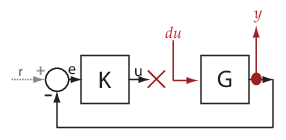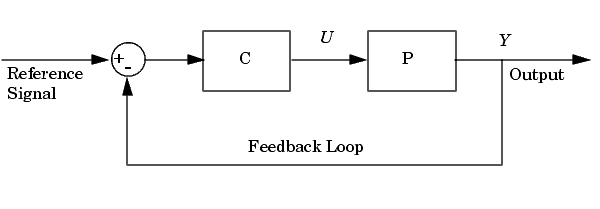Documentation

## Specify Portion of Model to Linearize

To linearize a subsystem, loop, or block in your model, you use analysis points. Each analysis point that you define in the model can serve one or more of the following purposes:

• Input — The software injects an additive input signal at an analysis point, for example, to model a disturbance at the plant input.

• Output — The software measures the signal value at a point, for example, to study the impact of a disturbance on the plant output.

• Loop Opening — The software interprets a break in the signal flow at a point, for example, to study the open-loop response at the plant input.

To compute a linear model for a portion of your system, specify a linearization input point and output point on the input and output signal to the portion of the model you want to linearize. To compute an open-loop response, specify loop openings to break the signal flow. You can also compute MIMO linear models by defining multiple input and output points.

### Analysis Points

You can specify the following types of linear analysis points using Simulink® Control Design™ software. These analysis points are pure annotations and do not impact model simulation.

Analysis PointDescription

Input perturbationSpecifies an additive input to a signal.

To define a transfer function for a linearized system, you can use an input perturbation with an output measurement or open-loop output.

For example, to compute the response G/(1+GK) in the example system, specify an input perturbation du and an output measurement y as shown.Output measurementTakes a measurement at a signal.

To define a transfer function for a linearized system, you can use an output measurement with an input perturbation or an open-loop input.

For example, to compute the response -K/(1+KG) in the example system, specify an output measurement point u and an input perturbation dy as shown.Loop breakSpecifies a loop opening.

Use a loop break to compute open-loop transfer function around a loop. Typically, you use loop breaks when you have nested loops or want to ignore the effect of some loops.

In the example system, the loop break stops the signal flow at u. As a result, the transfer function from the input perturbation de to the output measurement y is 0.Open-loop inputSpecifies a loop break followed by an input perturbation.

To linearize a plant or controller, you can use an open-loop input with an output measurement or an open-loop output.

For example, to linearize the plant in the example system, add an open-loop input before G and an output measurement y after G, as shown. The open-loop input breaks the signal flow at u, and adds an input perturbation du.Open-loop outputSpecifies an output measurement followed by a loop break.

To linearize a plant or controller, you can use an open-loop output with an input perturbation or an open-loop input.

For example, to compute the response -K in the example system, add an open-loop output after K and an input perturbation dy after G, as shown. The open-loop output breaks the signal flow and adds an output measurement u.Loop transfer functionSpecifies an output measurement before a loop break followed by an input perturbation.

To compute the open-loop transfer function around a loop, use a loop transfer analysis point.

For example, to compute -KG in the example system, specify the loop transfer analysis point as shown. The software adds an output measurement u breaks the signal flow, and adds an input perturbation du.Sensitivity functionSpecifies an input perturbation followed by an output measurement.

The sensitivity function measures how sensitive a signal is to an added disturbance. Sensitivity is a closed-loop measure. Feedback reduces the sensitivity in the frequency band where the open-loop gain is greater than `1`.

For example, to compute the sensitivity at the plant input of the example system, add a sensitivity function analysis point as shown. The software adds an input perturbation du followed by an output measurement u. The closed-loop transfer function from du to u is 1/(1+KG).Complementary sensitivity functionSpecifies an output measurement followed by an input perturbation.

The complementary sensitivity function at a point is the transfer function from an additive disturbance at the point to a measurement at the same point. In contrast to the sensitivity function, the disturbance is added after the measurement. Use this analysis point to compute the closed-loop transfer function around the loop.

For example, to compute the closed-loop transfer function for the example system, add a complementary sensitivity function analysis point as shown. The software adds an output measurement u followed by and input perturbation du. The closed-loop transfer function from du to u is -KG/(1+KG).### Opening Feedback Loops

If your model contains one or more feedback loops, you can choose to linearize an open-loop or a closed-loop system.

To remove the effects of a feedback loop, using analysis points lets you insert a loop opening without manually breaking the signal line. Manually removing the feedback signal from a nonlinear model changes the model operating point and produces a different linearized model. For more information, see How the Software Treats Loop Openings.

Proper placement of the loop opening is important for obtaining the linear model that you want. To understand the difference between open-loop and closed-loop analysis, consider the following single-loop control system.Suppose that you want to linearize the plant P about an equilibrium operating point of the model.

To linearize only the plant, you open the loop at the output of block P. If you do not open the loop, the linearized model between U and Y includes the effect of the feedback loop.

Loop open at Y?Transfer Function from U to Y
Yes

`$P\left(s\right)$`

No

`$\frac{P\left(s\right)}{1+P\left(s\right)C\left(s\right)}$`

The loop opening does not have to be in the same location as the linearization input or output point. For example, the following system has a loop opening after the gain on the outer feedback loop, which removes the effect of this loop from the linearization. As a result, only the blue blocks are on the linearization path.In this example, if you place a loop opening at the same location as the linearization output point, the effect of the inner loop is also removed from the linearization result.

### Ways to Specify Portion of Model to Linearize

There are several ways to define the portion of the model you want to linearize using linear analysis points. Each method has its own advantages and depends on which linearization tool you use. For more information on choosing linearization tools, see Choose Linearization Tools.

Specify portion of model...Use this method if...For more Information, see...
In Simulink modelYou want to save the analysis points directly in the model or graphically display the analysis points within the model.Specify Portion of Model to Linearize in Simulink Model
Using Linear Analysis ToolYou want to linearize your model interactively using the Linear Analysis Tool without changing the Simulink model. Using this method you can specify multiple open-loop or closed-loop transfer functions for your model.Specify Portion of Model to Linearize in Linear Analysis Tool
At command line using `linio` commandYou want to linearize your model using the `linearize` command. Using `linio` does not change the Simulink model.Specify Portion of Model to Linearize at Command Line
Using `slLinearizer` interfaceYou want to obtain multiple open-loop or closed-loop transfer functions from the linearized system without recompiling the model. Using this method does not change the Simulink model.Mark Signals of Interest for Batch Linearization
Using `slTuner` interfaceYou want to obtain multiple open-loop or closed-loop transfer functions from a tuned control system without recompiling the model. Using this method does not change the Simulink model.Mark Signals of Interest for Control System Analysis and Design
As a specific block or subsystemYou want to linearize a specific block or subsystem without defining analysis points for all the block inputs and outputs. Using this method does not change the Simulink model.Linearize Plant